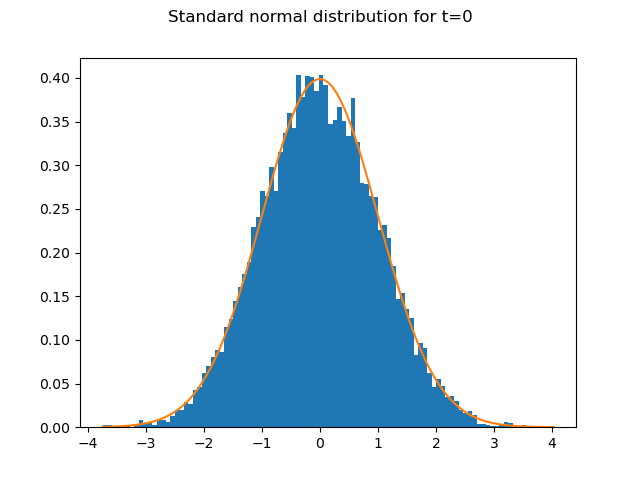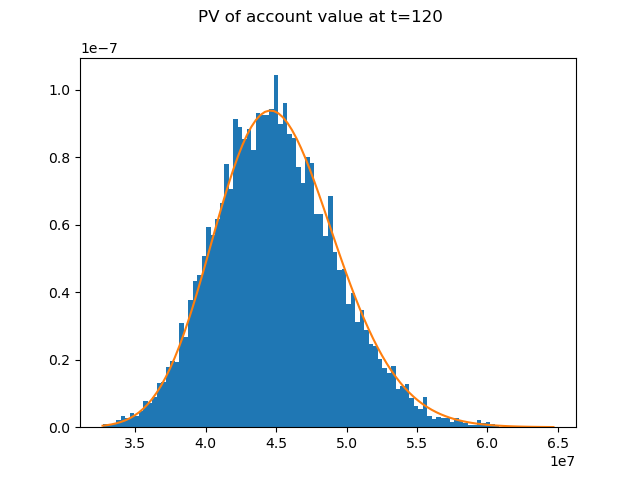# Account value distribution¶

10,000 random numbers drawn from the standard normal distribution are generated for each time step. The graph shows how well the 10,000 random numbers for t=0 fit the PDF of the standard normal distribution.

import modelx as mx
import pandas as pd
import matplotlib.pyplot as plt
from scipy.stats import norm, lognorm
import numpy as np

rand_nums = model.Projection.std_norm_rand()
pv_avs = model.Projection.pv_claims_from_av('MATURITY')
num_bins = 100
S0 = 45000000
sigma = 0.03
T = 10

fig, ax = plt.subplots()
n, bins, patches = ax.hist(rand_nums[:, 0], bins=num_bins, density=True)
ax.plot(bins, norm.pdf(bins), '-')
fig.suptitle('Standard normal distribution for t=0')The distibution of the account value at t=120 follows a log normal distribution. In the expression below, $$S_{T}$$ and $$S_{0}$$ denote the account value at t=T=120 and t=0 respectively.

$\ln\frac{S_{T}}{S_{0}}\sim\phi\left[\left(r-\frac{\sigma^{2}}{2}\right)T, \sigma\sqrt{T}\right]$

The graph shows how well the distribution of $$e^{-rT}S_{T}$$, the present values of the account value at t=0, fits the PDF of a log normal ditribution.

Reference: Options, Futures, and Other Derivatives by John C.Hull

fig, ax = plt.subplots()